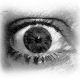Home > Grade 4 > Divisibility

# Divisibility

Directions: Using the digits 0 to 9 at most one time each, fill in the boxes to create a three-digit number. Try to create a three-digit number divisible by the greatest (or fewest) amount of the following factors: 2, 3, 4, 5, 6, 8, 9 or 10.

### Hint

What number should the final digit be such that it is divisible by 2, 5 and 10?

What should the three digits add up to so that it is divisible by 3 and 9?

What should the middle digit be so that it is divisible by 4?

Greatest number of factors:  360 and 720 are both divisible by 2,3,4,5,6,8,9 and 10.
Fewest number of factors:  any three digit prime with unique digits would have none of the given numbers as factors. There are more than 20 such numbers (e.g. 103, 347, 521, 849).

Source: Kelly Zinck

## Create A Pattern

Directions: Use the digits 0 to 9, at the most one time each, place a …

1.I believe the answer drop down box is wrong as 360 and 740 are divisible by 2, 3, 4, 5, 6, 8, 9, and 10. The six is missing form the drop down menu answer. Thanks for the cool problem.

••740 is NOT divisible by 3…or 6…or 8…or 9!!

•Problem states 720 not 740.

2.Never Telling My Name

you should put 180 in “2, 3, 4, 5, 6, 8, 9”

3.